## Regression Probability in Deep Networks (Bayesian Deep Learning Part II)

This post is a continuation of Explaining Dropout (Bayesian Deep Learning Part I). It is continued here for the classification problem.

Operations in the physical world are inherently uncertain, and the consequences of not understanding when to act upon the information you have are severe. Some of the most prevalent algorithms in robotics, such as particle filters for SLAM and Kalman filters for sensor fusion, are popular because they handle this uncertainty. And while no one is going to dispute the power of deep neural networks, now famous for tasks such as image classification, object detection, and text-to-speech, they do not have a well understood metric of uncertainty. You may have noticed this when Google maps happily sent you to Rome instead of Home.

The interpretation of dropout shown in the previous post casts the output of a neural network with dropout as an approximation to the output’s  true distribution. Since it is now a distribution instead of a point estimate, we can derive an expected value and variance.

The derivation for regression (taken from Yarin Gal’s thesis) is a little more straightforward, so I’ll go through that one first.

Expected Value

Since we are dealing with a probability distribution, we don’t get a single output – we must instead find the expected value of the distribution. The formula for an expected value of a continuous distribution is:Using our approximating distribution,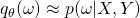, and the chain rule for probability, we get the integral:Whereis our approximating distribution,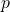is our actual distribution for input, label, and parameters (weights).andrepresent our training data. Note that since our weights are random variables, this becomes a double integral as we need to marginalize them out.

In the previous post, I mentioned that a probability prior would have to be found in order to make the objective functions match. It turns out that this prior amounts to placing independent normal distributions across each weight, with a specific standard deviation (). We can then reformulate:The expected value of a Gaussian distribution is just its mean, and the mean is determined by our network output (the parameter),. So if we integrate out our, we find the expected value with one more parameter to marginalize out.If we evaluate every possible combination of weights and average them (since the more likely output will occur more frequently), we can estimate this integral. This is called Monte Carlo Integration.

Since the weights are Bernoulli distributed, a combination of weights is simply one potential set of activations. Or, pragmatically, a forward pass with dropout enabled. Each of these forward passes results is a single Monte Carlo sample, which leaves us with the final estimator:

(1)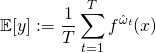For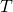samples. As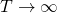, we approach the true expected value.

Variance

While performing inference time dropout improves network performance slightly, the  gain is not sufficient to justify performing multiple inferences for Monte Carlo integration. The real reason this process is valuable is its ability to estimate variance.

(2)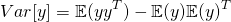I note that my version of the equation differs slightly from the one in Gal’s thesis. The only reason for this is that I more traditionally see covariance written asfor column vectors, while Gal is performs his derivation with row vectors.is known from previous, but we still need to figure out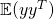. Conveniently, the procedure is much the same as for expected value. We start with the expected value formula, usinginstead of. We note thatandhave the same probability density function.We note that the integral with respect tois just the expected covariance, so we can rearrange 2 such that it represents expected variance.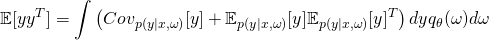Since we know, and the covariance, this becomes:The term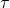is actually a characteristic of the network itself. It’s tied to the weight-decay parameter and dropout rate. I haven’t needed to dig my teeth into this yet, so I can’t provide a good explanation of that parameter. HOWEVER, the definition of this term is such that if your network has no weight decay parameter, it can be ignored (as,).

Since ourterm is not dependent on, andintegrates to 1, we can pull it out of the integral.  Monte Carlo integration is performed on the remaining term in the same manner as 1. This results in the final unbiased estimator for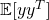: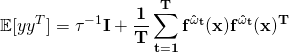And substituting this estimate back into 2 we end up with our final estimator for variance:## Explaining Dropout (Bayesian Deep Learning Part I)

Dropout is a now-ubiquitous regularization technique introduced by Hinton in 2012, and originally provided without any meaningful theoretical grounding. In a network with dropout, neurons are randomly turned on and off at training time, and the outputs are averaged at inference time. Intuitively, this creates a ensemble of classifiers, each of which focuses on slightly different features, thus preventing overfitting. This quora question explains it a bit more in depth in a few different ways.

While intuitively satisfying and generally accepted, this explanation of dropout lacks mathematical rigor. A Bayesian perspective, taken recently by Yarin Gal provides a mathematically grounded explanation of dropout, showing it is equivalent to minimizing the divergence between  true and approximate distributions over the network weights.

I’ve pulled the derivation that shows this for a single hidden layer network from his thesis, and added a bit of commentary based on my understanding of it.

Finding an Approximating Distribution

Withas our weights,our variational parameters, andandour training inputs and outputs, we find the best approximating distribution,, for our actual distribution,, by minimizing Kullback-Leibler divergence.   Or, in less words, we seek to minimize:Via the magic of Bayes’ theorem and logarithm rules, we can reach an alternate form of the loss: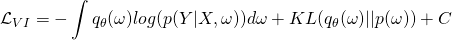We note that ourandare discrete, and reformat our log probability: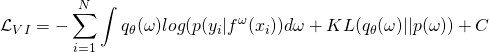Where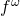represents the model output for a given input and weight parameters, and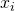andrepresent single inputs and outputs from our training set,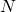is the number of training samples. As a constant,can (and will be) dropped from our optimization procedure.

Further, we note we can reformulate our final loss function using a subset of the training data:Where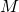is the size of our set. Theterm works as a normalizer, making sure our scale doesn’t change. This is important to show because dropout generates a sample population, not a predictable iteration through all datapoints.

Utilizing the pathwise derivative estimator, we arrive at a new loss function:whereis drawn from an as of yet undefined distribution.

Reformulating Dropout

We’ve reformulated our KL minimization, now we need to reformulate dropout to match. We start with a standard regularized loss function, whereandrepresent weights and biases with no dropout:In Consistent inference of probabilities in layered networks: Predictions and generalizations, it is shown that the squared error term is equivalent to: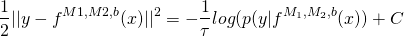If we designate a function, which corresponds to a dropout layer if the epsilon are a Bernoulli distribution, our fully formed loss function becomes:What this means

If you look closely, you can see how similarand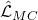are. Essentially, if we can select a prior,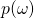, where the KL convergence is equal to our L2 penalty, they match to a scale.

Of course, due to the fact that it’s implemented via enabling and disabling neurons, we don’t ACTUALLY have to worry about this in implementation. But, mathematically, it turns out that by setting the prior to independent multivariate normals with appropriate variance, we can make this true.

Most importantly, though, this means that we can link mathematics related to the approximating distribution and KL estimation to our dropout, which becomes important when we talk about the idea of Bayesian Deep Learning (continued here if you want to read about regression, or here for classification).